150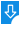10
2585

# Prime Counting Function Model  Free Download

Prime Counting Function Model Description:
Even if the trial division algorithms is one of the less efficient ones, it comes in handy when you need to to perform calculations. It's useful when a simulation plots and accumulates data from the parallel computation. Prime Counting Function Model allows you to select the number of independent threads as well as observe the computational time.Prime Counting Function Model: Prime Counting Function Model for Mac, A simulation that computes number of primes equal or less to the number N using the trial division algorithm.
Publisher: Wolfgang ChristianDownload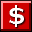Buy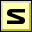Screenshot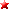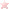Rate it
Category: Math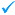Publisher: Wolfgang Christian    More titles >>
Last Updated: 01/25/2019
Requirements: Mac OS X